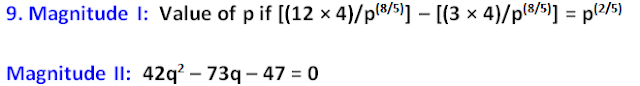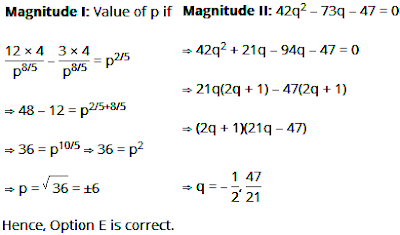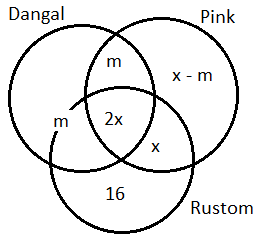# Quantitative Aptitude Practice Questions for IBPS Clerk – Set 8

Quantitative Aptitude Practice Questions for IBPS Clerk – Set 8
Directions (1 – 5): Each of the questions below consists of a question and two statements numbered I and II given below it. You have to decide whether the data provided in the statements are sufficient to answer the question. Read both the statements and give answer:
a) The data in statement I alone is sufficient to answer the question, while the data in statement II alone is not sufficient to answer the question.
b) The data in statement II alone is sufficient to answer the question, while the data in statement I alone is not sufficient to answer the question.
c) The data in statement I alone or in statement II alone is sufficient to answer the question.
d) The data in both the statements I and II is not sufficient to answer the question.
e) The data in both the statements I and II together is necessary to answer the question
1. Thirty percent of the banking aspirants are boys. Ten percent of the girl aspirants have the CCC certificates. What is the percentage of boy aspirants who have CCC certificate?
I. Twenty five per cent of the aspirants have CCC certificates.
II. Number of boy aspirants having CCC certificates are 20% more than the number of girl aspirants having CCC certificates.
2. Suresh Reddy has decided to take a non-stop flight from IGIA Delhi to Sydney in Australia. He is scheduled to leave Delhi at 5 am, as per Indian standard time on December 10, 2016. What is the local time at Sydney when he reaches there?
I.  The average speed of the plane is 700 km/hr.
II.  The flight distnace is 10,500 kilometers.
3. Is India's GDP higher than that of Australia?
I.  GDPs of India and Australia have grown over the past five years at compounded annual rate of 5% and 6% respectively.
II.  Five years ago, GDP of India was higher than that of Australia.
4. Akshay bought gold coins on a certain day, and sold them the next day. While buying and selling he had to pay to the broker one percent of the transaction value of the gold as brokerage. What was the profit earned by him per rupee spent on buying the gold coins?
I. The sales price per gold coin was 1.05 times that of its purchase price.
II. The number of gold coins purchased was 100.
5. Iyer family has only one kid. Mr. Iyer, the father says "after 'n' years, my age will be 4 times the age of my kid." Mrs. Iyer, the mother says "after 'n' years, my age will be 3 times that of my kid." What will be the combined ages of Mr. Iyer and Mrs. Iyer after 'n' years?
I.  The age difference between the Mr. and Mrs. Iyer is 10 years.
II.  After 'n' years the kid is going to be twice as old as she is now.
Directions (6 – 10): In each of the following questions, read the given statement and compare the Magnitude I and Magnitude II on its basis.
a) Magnitude I > Magnitude II
b) Magnitude I < Magnitude II
c) Magnitude I ≥ Magnitude II
d) Magnitude I ≤ Magnitude II
e) Magnitude I = Magnitude II or no relation can be established
6. Magnitude I: Subhas earned an average of Rs. 1500 per month from January through May. Then he earned Rs. 1600, Rs. 1700, Rs. 1800, Rs. 1900 and Rs. 2000 during the months from June through October. During November he earned 50% of what he earned in December. If his average earning for the entire year is Rs. 1500, then his earnings in the month of November.
Magnitude II: A certain sum compounded annually, at the rate of 20% gives the amount of 864 after 3 years. What was the principal 3 years ago.
7. Magnitude I: The probability that Ajay speaks truth is 3/5 and that of Varun speaking truth is 4/7. What is the probability that they agree in stating the same fact?
Magnitude II: Out of 10 people working on a project, 4 are skilled. If 3 are selected, what is the probability that there is at least one skilled among them?
8. Magnitude I: In a right angled triangle ABC, find the height of the altitude BD drawn from B to the hypotenuse AC; given AB = 9 cm and BC = 12 cm.
Magnitude II: A fruit seller bought 600 guava at Rs. 30 per 20 guavas. 250 guavas got spoiled and hence thrown He sold 24 dozen guavas at the rate of Rs. 30 per dozen and the remaining at Rs. 2.5 per guava, then the total profit/loss10. Magnitude I: In a group of 80 children and 10 youngster, each child got candies that are 15% of the total number of children and each youngster got candies that are 25% of the total number of children. How many candies were there?
Magnitude II: Vijay and Shekhar started a business with investments of Rs. 20k and 24k respectivley. At the end of one year, the total profit they shared was Rs. 1000 more than the difference in their profit share, then the total profit
Directions (11 – 12): Study the following information and asnwer the questions that follow:
Three popular movies, Dangal, Pink and Rustom, were nominated for the best film award. 60 persons were asked which film deserved the award the most.
16 people thought only Rustom deserved it.
The number of people who thought that all the three films equally deserved the award was twice the number of people who thought that Pink and Rustom deserved that award but Dangal did not.
The number of people who thought that Pink deserved that award was equal to twice the number of people who thought all the three equally deserved it.
The number of people who thought that Dangal and Pink, but not Rustom deserved the award was equal to the number of people who thought that only Dangal or Dangal and Rustom but not Pink deserved it.
11. Find the maximum number of people who thought that all the three movies deserved the award?
a) 36
b) 22
c) 44
d) 21
e) None of these
12. Find the number of people who thought that Pink deserved the award?
a) 44
b) 32
c) 22
d) 11
e) None of these
Directions (13 – 15): Study the following information carefully and answer the question given beside:
Export value of three materials and thier percentage share
 Product 2015-16 2016-17 Plastic 23% Cotton Mineral oils 26% Total value in crore (₹) 22400 25800
I.  In 2015-16, the value of export of cotton exceeds that value of Mineral Oils by 100%
II.  In 2016-17 the ratio of the % value of export of Mineral oils to that of Cotton is 3 : 4.
III.  The export value of Plastic in 2016-17 exceeds its value in 2015-16 by Rs. 1006 crore.
13. The export value of Mineral oils during 2015-16 was approximately how much per cent less than that of during 2016-17?
a) 32%
b) 24%
c) 38%
d) 43%
e) 28%
14. What is the percentage change in the value of export of Cotton from 2015-16 to 2016-17?
a) 2.2
b) 2.9
c) 2.5
d) 4.6
e) 3.2
15. During 2015-16, how much more is the value of export of Mineral oils than that of Plastic?
a) Rs.886 cr
b) Rs. 896 cr
c) Rs.906 cr
d) Rs.856 cr
e) Rs.962 cr

Solutions:
1. C) Let there be 100 aspirants, So, as per the question, 30 among them will be boys and the rest 70 will be girls.
10% of 70 = 7 girl aspirants have CCC certificates.
From Statement I: 25% aspriants have CCC certifiactes = 25% of 100 = 25
25 – 7 = 18 boy aspirants have CCC certificates.
Reqd % = 18/30 × 100
Hence, Statement I alone is sufficient.
From Statement II:
Number of boy aspirants having CCC certificates = 1.2 × 7
Reqd % = (1.2 × 7)/30 × 100
Hence, statement II is also suffcient.
Hence, option C is correct
2. D) From the information in statment I and II alone we can't determined the answer.
From statement I and II: By combining both the statments we can get the duration of the flight. But for the arrival time we should have the information regarding the time zone difference of Delhi and Sydney.
Hence, option D is correct.
3. D) From statement I: This statement does not give us the actual values of their GDP's 5 years ago.
From Statement II: We know that GDP of India is greater than that of Australia but we do not know by how much is greater.
Even after combining both the statements the question can't be answered.
Hence, option D is correct.
4. A) From Statement I:  The cost of buying the gold coins for Akshay is
CP + 0.01 CP = 1.01 CP
The cost of selling the gold coins for Akshay is
SP – 0.01 SP = 0.99 SP
Profit = Cost of selling – Cost of buying  = 0.99 SP – 1.01 CP
SP = 1.05 CP
Profit = (0.99 × 1.05) CP – 1.01 CP = 0.0295 CP
Profit earned per rupee spent on buying the gold coins is Rs. 0.0295.
From the number of gold coins given in the statement II, we can't conclude anything.
Hence, option A is correct.
5. A) Let the present age of father (Mr. Iyer) = f years
The present age of mother (Mrs. Iyer)= m years
The present age of kid = k years
f + n = 4(k + n)          ..(i)
m + n = 3 (k + n)     ...(ii)
Eqn. (i) and (ii), we get
f – m = k + n     ....(iii)
Eqn. (i) + Eqn. (ii)
f + m + 2n = 7k + 7n = 7(k + n)    ....(iv)
From statement I:
f – m = 10
Substituting in eqn. (iii), we get
k + n = 10
The combined age of the Mr. and Mrs. Iyer after n years = 7 (k + n)
= 7 × 10 = 70 years
Statement I alone is sufficient.
From statement II:
k + n = 2k
n = k       ....(v)
Substituting eqn. (v) in equation (iii), we get
f – m = 2n
Substituting eqn. (v) in equation (iv), we get
f + m = 14n – 2n
f + m = 12n
The value of n is not known.
We can't find the combined age of parents.
Statement II alone is not sufficient.
Hence, option A is correct.
6. E) Magnitude I: Let the earnings for the month of November be x. Then the earnings in December will be 2x.
Now, the total annual earnings = (1500 × 5) + 1600 + 1700 + 1800 + 1900 + 2000 + x + 2x = 16500 + 3x
Total earnings for the given year = 12 × 1500 = Rs. 18000 which is equal to 16500 + 3x
16500 + 3x = 18000 3x = 1500 x = 500 rupees
Magnitude II: Amount = 864, Rate of interest = 20%
Calculate the net% effect for 3 years @ 20%, applying the net% effect formula
Net % effect = x + y + (xy/100)%
For first 2 years, here x = 20% and y = 20%
= 20 + 20 + (20 × 20)/100 = 44%
For 3rd year, here x = 44% and y = 20%
= 44 + 20 + (44 + 20)/100 = 64 + 8.8 = 72.8%
From here, we can conclude that (100 + 72.8)% = 864
Let the principal be x
172.8% = 864
100% = x
x = (864 × 100)/172.8 = 500
Hence, Magnitude I = Magnitude II
7. B) Magnitude I: If both agree stating the same fact, either both of them speak the truth or both speak false.
Probability = 3/5 × 4/7 + 2/7 × 3/7 = 12/35 + 6/35 = 18/35
Magnitude II: P(at least one skilled person) = 1 – P(no skilled person)
= 1 – (6C3)/(10C3) = 1 – [(6 × 5 × 4)/(10 × 9 × 8)] = 5/6
Hence, Magnitude I < Magnitude II
8. B) Magnitude I: Since the sides containing the right angle are 9 cm and 12 cm, the area of te triangle = 1/2 × 9 × 12 = 54 sq.cm
As AB = 9 cm, BC = 12 cm, AC will be 15 cm. Considering AC as the base and BD as the altitude, the area of ΔABC = 1/2 × AC × BD = 1/2 × 15 × BD = 54
BD = (54 × 2)/15 = 108/15 = 7.2cm
Magnitude II: Cost price of guavas = 600 × 30/20 = Rs.900
Remaining guavas = 600 – 250 = 350
Selling price of guavas sold at Rs. 30 per dozen = 24 × 30 = Rs. 720
Selling price of guavas sold at Rs. 2.5 per guava
= (350 – 24 × 12) × 2.5
= (350 – 288) × 2.5 = 62 × 2.5 = Rs. 155
Total selling price = 720 + 155 = 875
Total loss = 900 – 875 = 25
Hence, Magnitude I < Magnitude II
9. E)10. A) Magnitude I: Number of children = 80
Number of youngesters = 10
Number of candies each child got = 15% of 80 = 15/100 × 80 = 12
Number of candies 80 children got = 80 × 12 = 960
Number of candies each youngster got = 25% of 80 = 20.
Number of candies 10 youngers got = 10 × 20 = 200.
Total number of candies = 960 + 200 = 1160.
Magnitude II: Ratio of profits of Vijay and Shekhar = Ratio of investments = 5 : 6
Let the profit shares of Vijay and Shekhar be 5x and 6x respectively.
6x + 5x = 6x – 5x + 1000 x = 100
Total profit = 11x = Rs. 1100
Hence, Magnitude I > Magnitude II
(11 – 12): Explanation:  Let x be the number of people who thought that Pink and Rustom deserved the award but Dangal did not.
Then the number of people who thought all three equally deserved it is 2x.
Let m be the number of people who thought that Dangal and Pink, but not Rustom deserved the award.
The number of people who thought that Pink deserved = 4x
The number of people who thought that only Pink deserved the award = 4x – (m + 2x + x) = x – m
From the given data, we can draw the following Venn diagram.Thus, 2m + x – m + 2x + x + 16 = 60
m + 4x = 44
x is maximum when m = 0
x = 11
2x = 22
The maximum number of people who thought that all the three movies deserved the award = 22.
And, The number of people who thought that Pink deserved the award = 4x = 4 x 11 = 44
11. B) The maximum number of people who thought that all the three movies deserved the award = 22
12. A) The number of people who thought that Pink deserved the award = 44
(13 – 15): Common Explanation: As per the given information, we get
% export of cotton = 26 × 2 = 52% = 11648
% export of Mineral oils = 26% = 5824
% export of Plastic = (100 – 26 – 52)% = 22% = 492
 Product 2015-16 2016-17 Plastic 4928 (22%) 5934(23%) Cotton 11648(52%) 11352(44%) Mineral oils 5824 (26%) 8514(33%) Total value in crore (₹) 22400 (100%) 25800 (100%)
13. A) Reqd % = (8514 – 5824)/8514 × 100 = 31.6% ≈ 32%
14. C) Percentage change in the value of export of cotton from 2015-16 to 2016-17
= (11648 – 11352)/11648 × 100 = 2.5%
15. B) Following the common explanation, we get, In 2015-16,
Reqd difference = 5824 – 4928 = ₹ 896 Cr